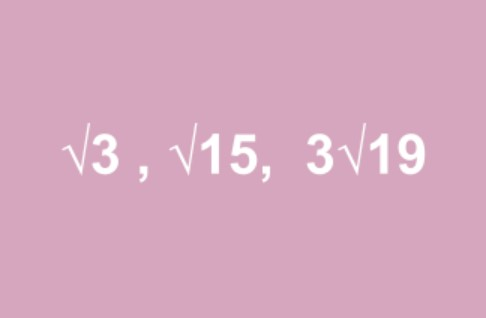# Surd Form Calculator

To know if a number is surd or not, enter it in the surd form calculator.

Give Us Feedback

## Surd Form Calculator

Surds calculator/simplifier is a mathematical tool related to roots. It is devised to tell if a root is surd or not.

## How to use the surd calculator?

1. Input the root value e.g 4√.
2. Input the number e.g √78.
3. Press “Enter”

The calculator will tell the result. Reset the tool to use again.

## What is a surd?

Such a whole number, which when solved for its under root, does not give an exact value. For example:In other words, surds are such square roots that give irrational decimal values on simplifying.

## How to identify the surds?

To identify the surd numbers you need to simplify them. If a root on simplification gives a rational number then it is not a surd and vice versa.

You can use the surd form calculator to avoid simplification.

Example:

Tell whether these numbers are surds or not.

√35, 50, 5√243

Solution:

1. √35 = 5.9160… (irrational) so it is a surd.
2. 50 = 7.0710… (irrational) it is a surd.
3. 5√243 = 3 (rational) it is not a surd.

See more surd examples in the table below.

 Number Simple form Decimal form Is it Surd? √2 √2 1.4142135... Surd √3 √3 1.7320508... Surd √4 2 2 Not a surd √¼ ½ 0.5 Not a surd 3√11 3√11 2.2239800... Surd 3√27 3 3 Not a surd 5√3 5√3 1.2457309... Surd

### Math Tools